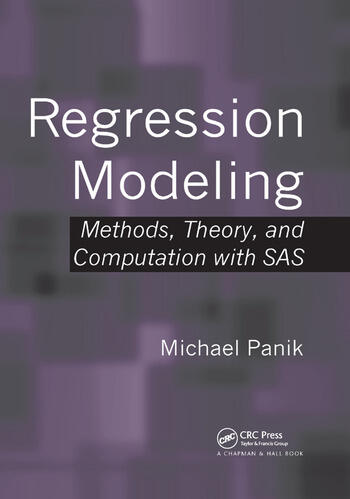# Regression Modeling: Methods, Theory, and Computation with SAS

## 1st Edition

Michael Panik

Chapman and Hall/CRC
Published October 7, 2019
Reference - 830 Pages - 94 B/W Illustrations
ISBN 9780367385675 - CAT# K450471

For Instructors Request Inspection Copy

was \$74.95

USD\$59.96

SAVE ~\$14.99

FREE Standard Shipping!

## Preview

### Summary

Regression Modeling: Methods, Theory, and Computation with SAS provides an introduction to a diverse assortment of regression techniques using SAS to solve a wide variety of regression problems. The author fully documents the SAS programs and thoroughly explains the output produced by the programs.

The text presents the popular ordinary least squares (OLS) approach before introducing many alternative regression methods. It covers nonparametric regression, logistic regression (including Poisson regression), Bayesian regression, robust regression, fuzzy regression, random coefficients regression, L1 and q-quantile regression, regression in a spatial domain, ridge regression, semiparametric regression, nonlinear least squares, and time-series regression issues. For most of the regression methods, the author includes SAS procedure code, enabling readers to promptly perform their own regression runs.

A Comprehensive, Accessible Source on Regression Methodology and Modeling
Requiring only basic knowledge of statistics and calculus, this book discusses how to use regression analysis for decision making and problem solving. It shows readers the power and diversity of regression techniques without overwhelming them with calculations.

#### Instructors

We provide complimentary e-inspection copies of primary textbooks to instructors considering our books for course adoption.